@evilking 2018-05-01T11:12:08.000000Z 字数 6012 阅读 1751

时间序列篇

# ARIMA模型

## $ARIMA$ 模型的结构

• $d=0$ 时，$ARIMA(p,d,q)$模型实际上就是 $ARMA(p,q)$ 模型.

• $p = 0$ 时，$ARIMA(0,d,q)$模型可以简记为 $IMA(d,q)$ 模型.

• $q = 0$ 时，$ARIMA(p,d,0)$ 模型可以简记为 $ARI(p,d)$ 模型.

• $d = 1,p = q = 0$ 时，$ARIMA(0,1,0)$模型为:

该模型称为随机游走(random walk)模型，或醉汉模型.

## $ARIMA$模型的性质

### 平稳性

$\varphi(B) = \Phi(B) \nabla^d$$\varphi(B)$ 称为广义自回归系数多项式.显然 $ARIMA$ 模型的平稳性完全由 $\varphi(B) = 0$的根的性质决定.

### 方差齐性

$Var(x_t) = Var(x_0 + \epsilon_t + \cdots + \epsilon_1) = t \sigma_{\epsilon}^2$

$1$ 阶差分之后

## $ARIMA$ 模型建模

$ARMA$ 模型差分运算yesnoyesno

#数据的准备> d <- read.table("data/file17.csv",",",header = T)> x <- ts(d$index,start = 1952)> plot(x)>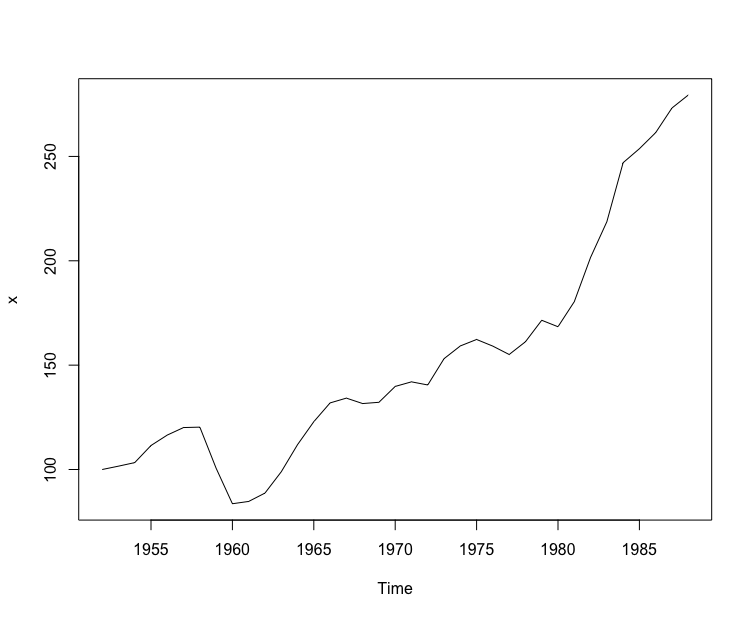这里是对 1952 ~ 1988 年中国农业实际国民收入指数序列建模. #一阶差分> x.dif <- diff(x)> plot(x.dif)>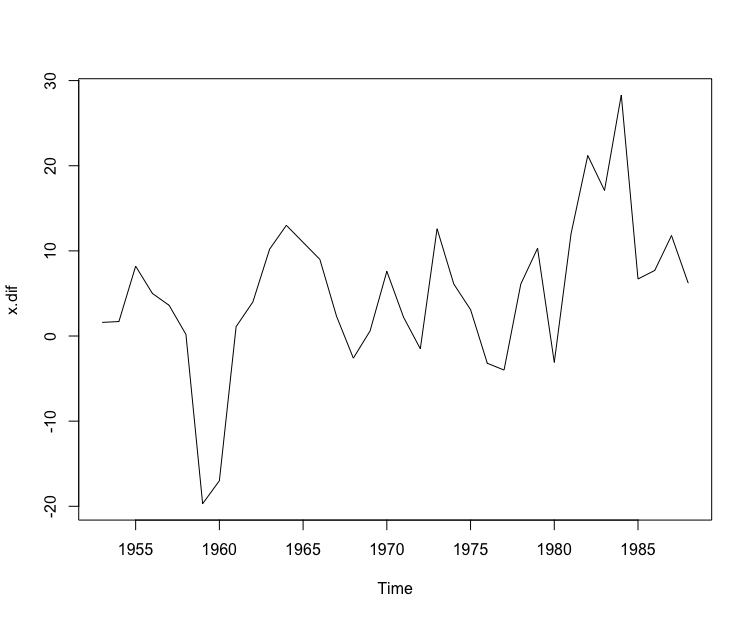一阶差分后可以看到时序图基本平稳，不用进行二阶差分. #考察自相关图> acf(x.dif)>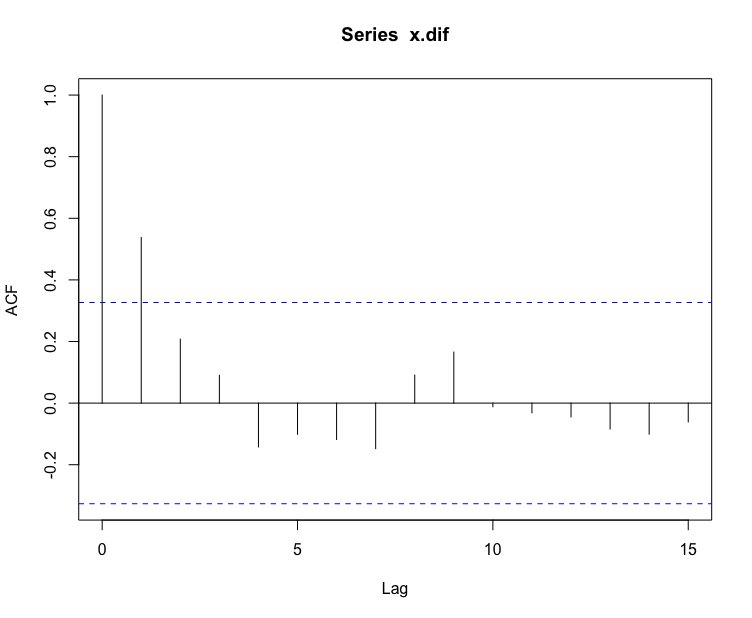从自相关图中可以看出，一阶滞后后的自相关阶数均在 2 倍标准差范围之内，显示出很强的短期相关性，所以可以认为 1 阶差分后序列平稳，相关系数 1 阶截尾. #考察偏自相关图> pacf(x.dif)>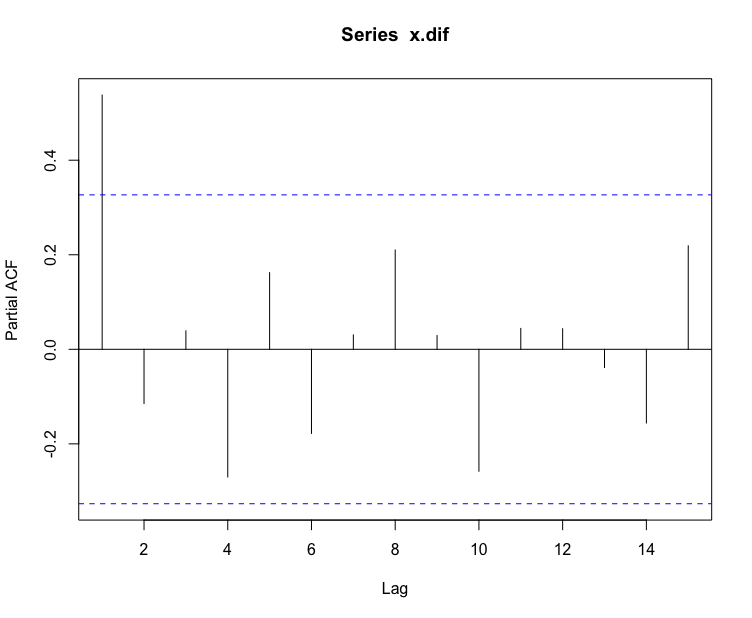从偏自相关图中看出，0 阶滞后后，偏相关阶数均在 2 倍标准差范围之内，偏自相关系数 0 阶截尾. 综合acfpacf，确定模型为 $arima(0,1,1)$. # arima(0,1,1) 模型拟合> x.fit <- arima(x,order = c(0,1,1))> x.fitCall:arima(x = x, order = c(0, 1, 1))Coefficients: ma1 0.7355s.e. 0.1545sigma^2 estimated as 61.95: log likelihood = -125.74, aic = 255.49> Coefficients参数值可以得到拟合模型的表达式为 #残差白噪声检测，考察拟合效果> for(i in 1:2) print(Box.test(x.fit$residuals,lag = 6*i))    Box-Pierce testdata:  x.fit$residualsX-squared = 3.3169, df = 6, p-value = 0.7681 Box-Pierce testdata: x.fit$residualsX-squared = 6.0284, df = 12, p-value = 0.9146>

## $ARIMA$ 模型预测

$ARIMA(p,d,q)$ 模型的一般表示方法为:

$ARMA$模型一样，也可以用随机扰动项的线性函数表示:

$l$ 期预测误差为:

$l$ 期预测误差的方差为:

#引入必要的时间序列预测包> library(zoo)> library(forecast)# arima 模型拟合> d <- read.table("data/file17.csv",",",header = T)> x <- ts(d\$index, start = 1952)> x.fit <- arima(x,order = c(0,1,1))# 模型预测> x.fore <- forecast(x.fit,h = 10)> x.fore     Point Forecast    Lo 80    Hi 80    Lo 95    Hi 951989       283.0082 272.9213 293.0950 267.5816 298.43471990       283.0082 262.8045 303.2118 252.1094 313.90701991       283.0082 256.2756 309.7407 242.1243 323.89211992       283.0082 251.0540 314.9624 234.1384 331.87791993       283.0082 246.5731 319.4432 227.2856 338.73081994       283.0082 242.5860 323.4304 221.1878 344.82861995       283.0082 238.9582 327.0581 215.6396 350.37671996       283.0082 235.6073 330.4090 210.5149 355.50151997       283.0082 232.4782 333.5382 205.7292 360.28711998       283.0082 229.5318 336.4845 201.2231 364.7932# 绘制预测图> plot(x.fore)>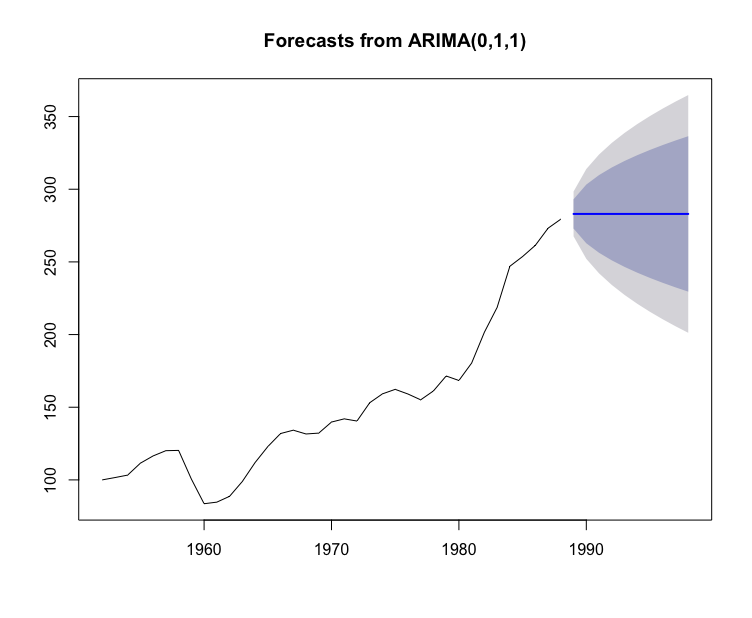arima()函数用来拟合模型，forecast()函数利用拟合好的模型来进行预测，具体的用法可以使用 help(arima)以及 help(forecast)命令查看帮助文档.

$ARIMA(p,d,q)$模型中，如果有部分系数缺省了，那么该模型称为疏系数模型，记为 $ARIMA((p_1,\cdots,p_m),d,(q_1,\cdots,q_n))$，其中 $p_1,\cdots,p_m$，以及$q_1,\cdots,q_n$分别表示自相系数的非零阶数和移动平滑部分的非零阶数.• 私有
• 公开
• 删除